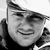Announcements
Applications are open for the 2024 Qlik Luminary Program. Apply by December 15 here.
cancel
Showing results for
Did you mean:Partner - Creator

## Set analysis month does not work

Hi everyone,

I'm struggling with set analysis. I would appreciate some help 🙂

I have this KPI which counts number of connections for actual month:

Also this one for previous month:

They both show correct values without filters or with filters no related to date (see attached capture 1), but if I use the "date picker filter" picking for example 3 days from October (let's say 23, 24, 25) it shows the correct value for those 3 days from actual month but not for those 3 days from previous month which shows "0" (see attached capture 2).

I would need that if I pick some days from any month (for example 23, 24, 25th of October), the previous month KPI shows values for 23,  24, 25th of September (currently it shows "0" as value).

Could anyone give me a clue?

Thanks to everyone.

Labels (4)

• ### setAnalysis

1 Solution

Accepted SolutionsCreator III

For actual month:

``count({<date_id={">=\$(=min(date_id)) <=\$(=max(date_id))"}*{">=\$(=num(monthstart(max(date_id)))) <=\$(=max(date_id))"}>}connect_time)``

In this set analysis, we make intersection of two different data set. First one is available data range (Min Date to Max Date) and the second one is Actual Month Date Range (month start of max date to Max Date). If you select a date range, It only shows values for the dates which are in Actual Month.

For Previous Month:

``count({<date_id={">=\$(=num(addmonths(min(date_id),-1))) <=\$(=num(addmonths(max(date_id),-1)))"}*{">=\$(=num(monthstart(max(date_id),-1))) <=\$(=num(addmonths(max(date_id),-1)))"}>}connect_time)``

Same logic for previous month.

You can modify this expressions according to your need.

Hope it helps..

5 RepliesPartner - Creator
AuthorMVP

Try to clear out all calendar selections in your set expressions like I've done below.

=count({\$<Date, Year, Week, YearMonth, Quarter, Month={"\$(=num(month(addmonths(max(date_id),0)),'00'))"}>}connect_time)

=count({\$<Date, Year, Week, YearMonth, Quarter, Month={"\$(=num(month(addmonths(max(date_id),-1)),'00'))"}>}connect_time)Partner - Creator
Author

Hi Vegar,

Thank you for your help. Doing that it ignores the days of the month I select (23, 24, 25th of October) and shows always the value for the whole month independently of the filters. But I don't look for that.

What I need is that if I pick 3 days of October (23, 24, 25th) the KPI for previous month shows values for 23, 24, 25th of September (not the whole month).Creator III

For actual month:

``count({<date_id={">=\$(=min(date_id)) <=\$(=max(date_id))"}*{">=\$(=num(monthstart(max(date_id)))) <=\$(=max(date_id))"}>}connect_time)``

In this set analysis, we make intersection of two different data set. First one is available data range (Min Date to Max Date) and the second one is Actual Month Date Range (month start of max date to Max Date). If you select a date range, It only shows values for the dates which are in Actual Month.

For Previous Month:

``count({<date_id={">=\$(=num(addmonths(min(date_id),-1))) <=\$(=num(addmonths(max(date_id),-1)))"}*{">=\$(=num(monthstart(max(date_id),-1))) <=\$(=num(addmonths(max(date_id),-1)))"}>}connect_time)``

Same logic for previous month.

You can modify this expressions according to your need.

Hope it helps..Partner - Creator
Author

Thank you Kaanerisen! You made my day 🙂Tags
Community Browser